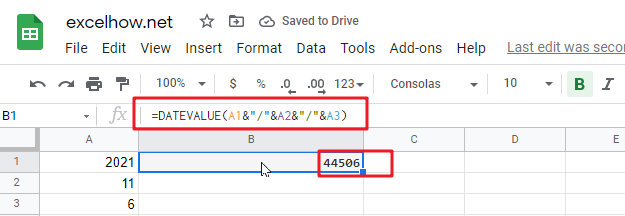This post will guide you how to use Google Sheets DATEVALUE function with syntax and examples.

## Description

The Google Sheets DATEVALUE returns the serial number of date and it can be used to convert a date represented as text format into a serial number that recognizes as a date format.

The DATEVALUE function can be used to convert a given date string in a known format to a date value. The purpose of this function is to convert a date in text format to a valid date and its returned values is a date value.

For example, you can convert a date “2021/11/6” that is text format to a serial number, you can use the formula: =DATEVALUE (“2021/11/6”). Then it returns a serial number “44506” you can use the google sheets dates to manipulate with formulas or pivot table to filter, sort. It is very useful than the text dates.

The DATEVALUE function is a build-in function in Google Sheets and it is categorized as a Date function.

## Syntax

The syntax of the DATEVALUE function is as below:

=DATEVALUE(date_text)

Where the DATEVALUE function arguments is:

• Date_text -This is a required argument. A valid date that the format is text. Or the text that represents a date in an excel date format.

Note:

• The date_text argument must represent a date between January 1, 1900 and December 31, 9999. The DATEVALUE function returns the #VALUE! Error value if the value of the date_text argument falls outside of this range.
• If the year portion of the date_text argument is omitted, the DATEVALUE function uses the current year from your computer’s built-in clock. Time information in the date_text argument is ignored.
• January 1 1900 is serial number 1 by default.
• Date_text argument must be a string, if a number or cell reference to a cell containing a number is provided, the DATEVALUE function will return the #VALUE! Error.
• If you want to provide an explicit string input to DATEVALUE function rather than a cell reference, you must surround quotation marks for inputting string.

## Google Sheets DATEVALUE Function Examples

The below examples will show you how to use google sheets DATEVALUE Function to return a serial number of a given date.

1# Get a serial number of a date (11/06/2021) the format as text, enter the following formula in Cell B1.

`=DATEVALUE("11/06/2021")`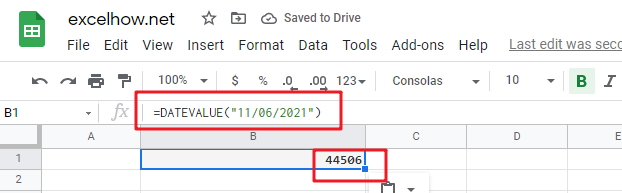2# Get a serial number of a date (6-Nov-2021) the format as text, enter the following formula in Cell B2.

`=DATEVALUE("6-Nov-2021")`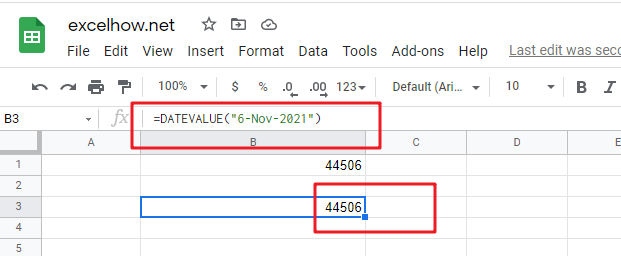3# Get a serial number of a date (2021/03/14) the format as text, enter the following formula in Cell B3.

`=DATEVALUE("2021/11/6")`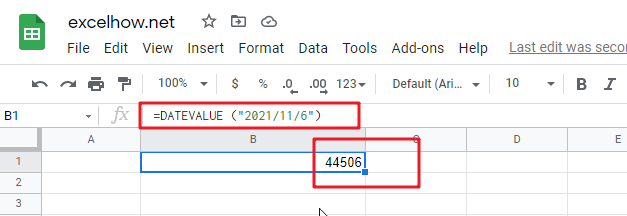4# get a serial number from a date that the year portion is omitted (11/6) assuming the current year is 2021, using the following formula:

`=DATEVALUE("11/6")`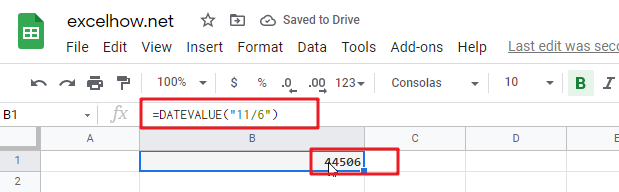5# get a serial number from a date that created by combining the values in Cells A1, A2, and A3

`=DATEVALUE(A1&"/"&A2&"/"&A3)`